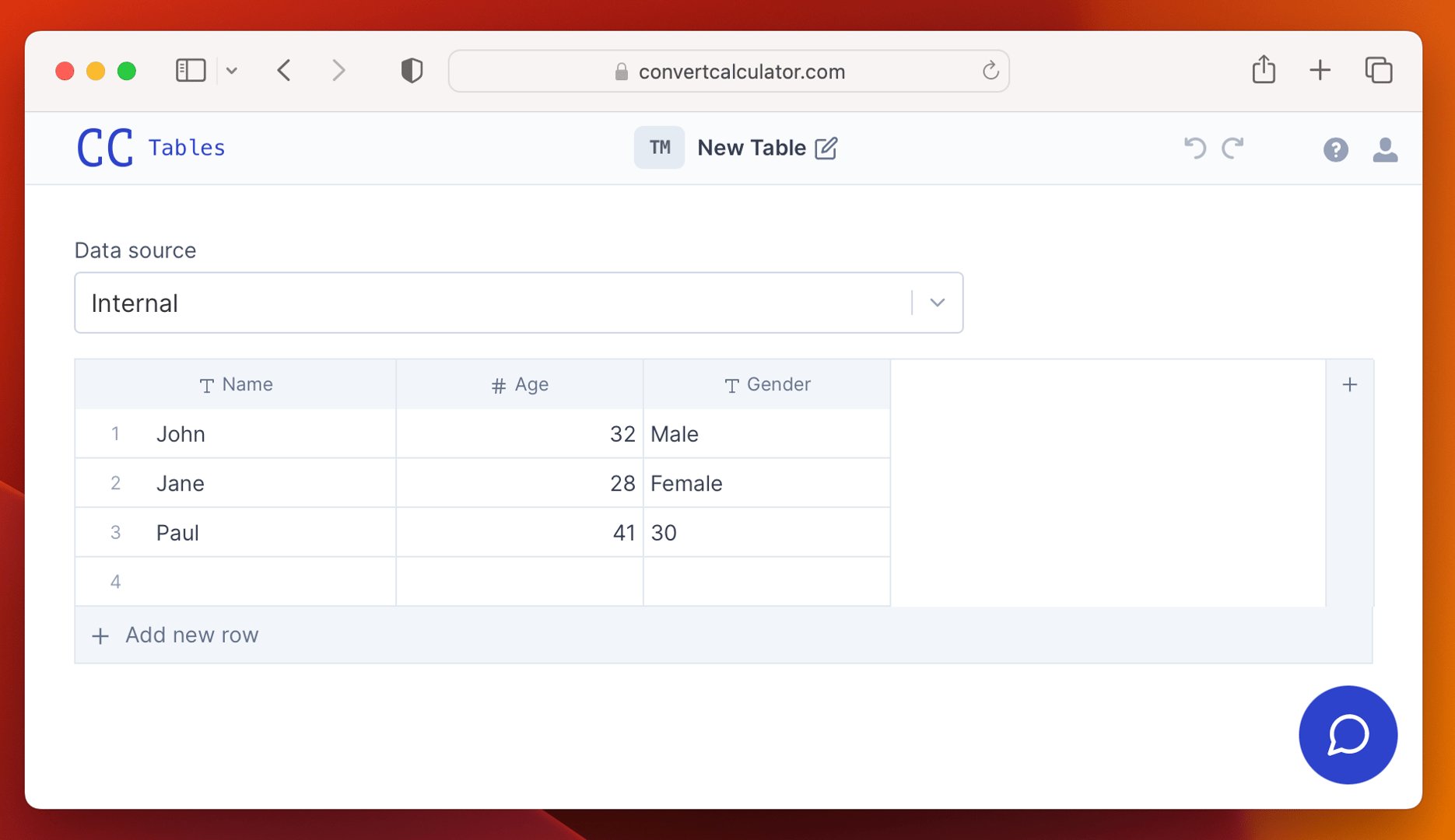# INDEX function

The INDEX function is a versatile function that allows you to return a value from a table or range based on the row and column numbers you specify.

The INDEX function has the following syntax:
`FS`INDEX(reference, row_num, col_num)``

Where:

• `reference` is a table, datasheet, or an array that you want to select a value from.
• `row_num` is the row number in the reference range from which you want to return a value. If the reference is a table or datasheet, `row_num` is the number of the row in the range. If the reference is an array, `row_num` is the position of the row in the array.
• 'col_num' is the column number in the reference range from which you want to return a value. If the reference is a table or datasheet, `col_num` is the number of the column in the range. If the reference is an array, `col_num` is the position of the column in the array.

For example, suppose you have a table TM, as shown below:To return the value in cell A2 (i.e., "Jane"), you could use the following INDEX function:

`INDEX(TABLE(TA), 2, 0)`

This function returns the value in the second row and first column of the Table.

Note: column indexes start counting at 0. If you find this confusing, you can also use A, B, C, etc. Just hover over the column header to see which letter applies to the column in your Table.

You can also use the INDEX function to return a value from a one-dimensional array. For example, to return the second value in the array {1,2,3,4}, you could use the following INDEX function:

`INDEX( ARRAY(1, 2, 3, 4, "five"), 4)`

or

`INDEX( ARRAY(1, 2, 3, 4, "five"), E)`

This function returns the value in the fifth position of the array, "five".

## Related articles